Ex 6.3

Chapter 6 Class 12 Application of Derivatives
Serial order wise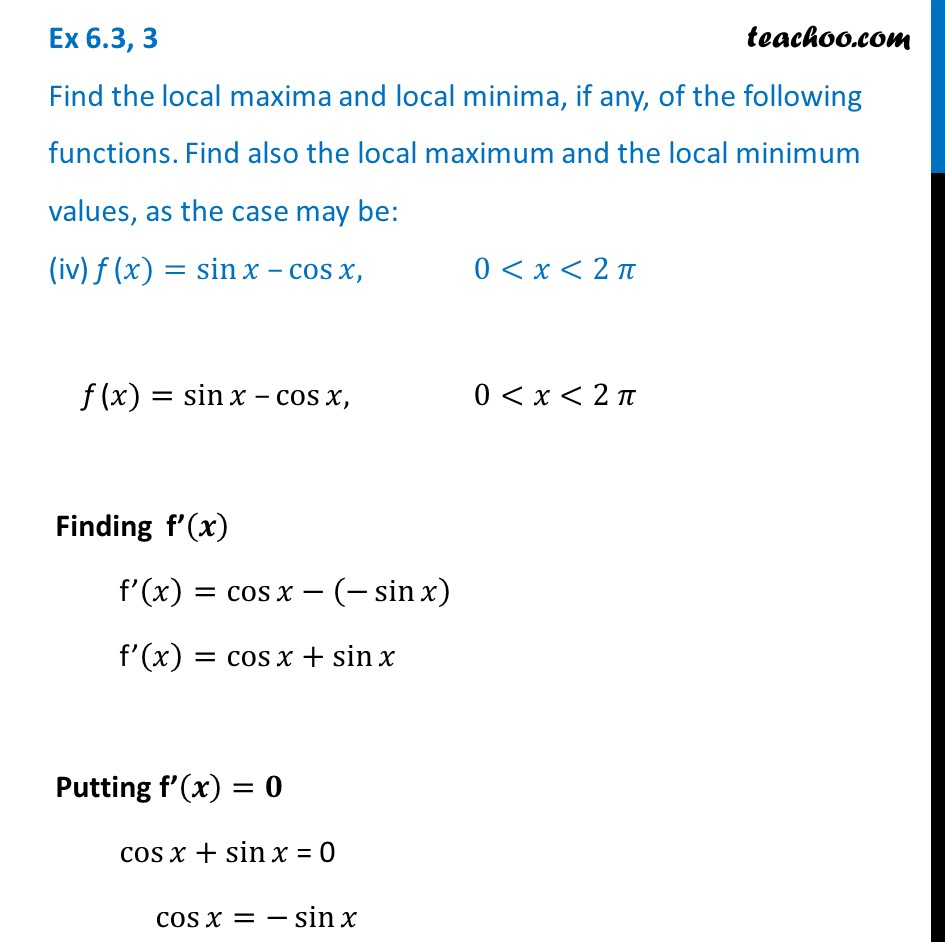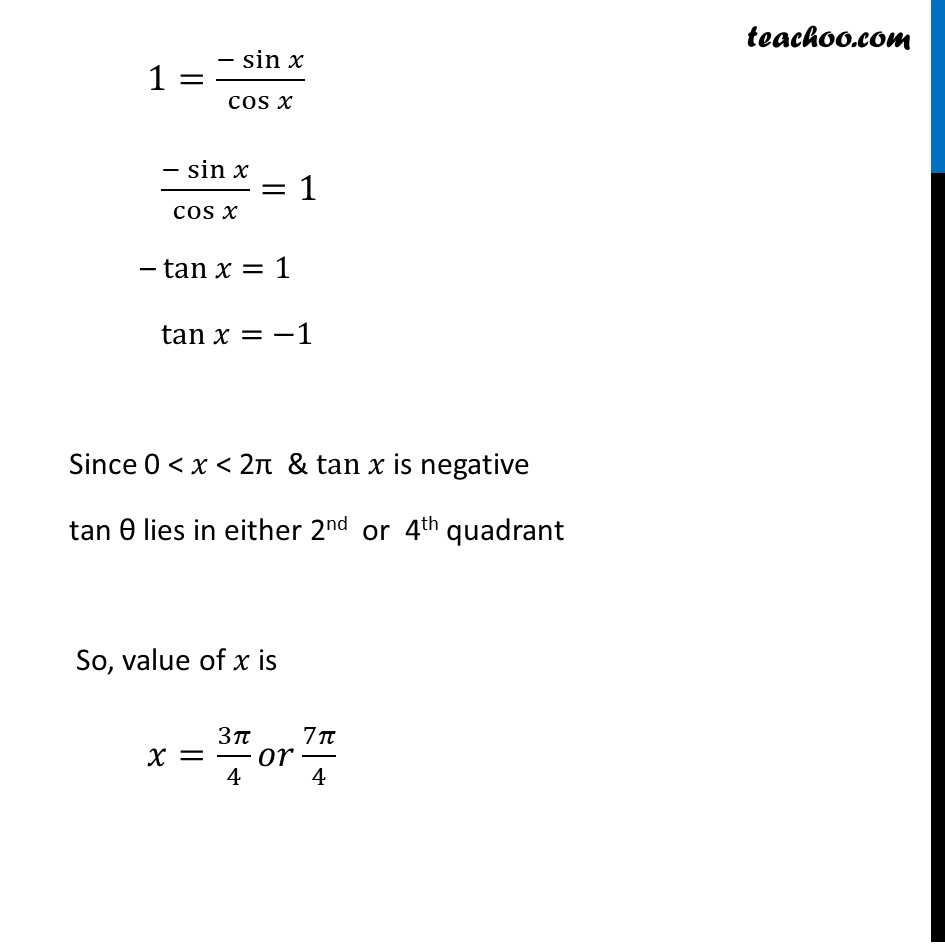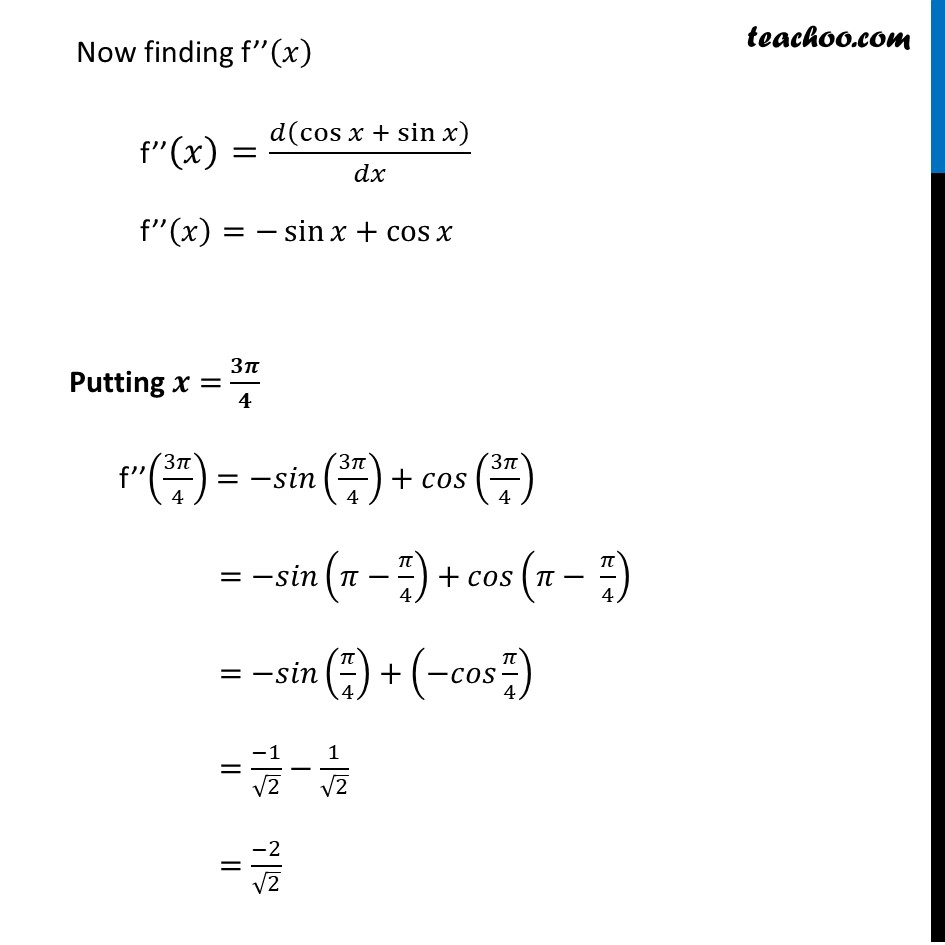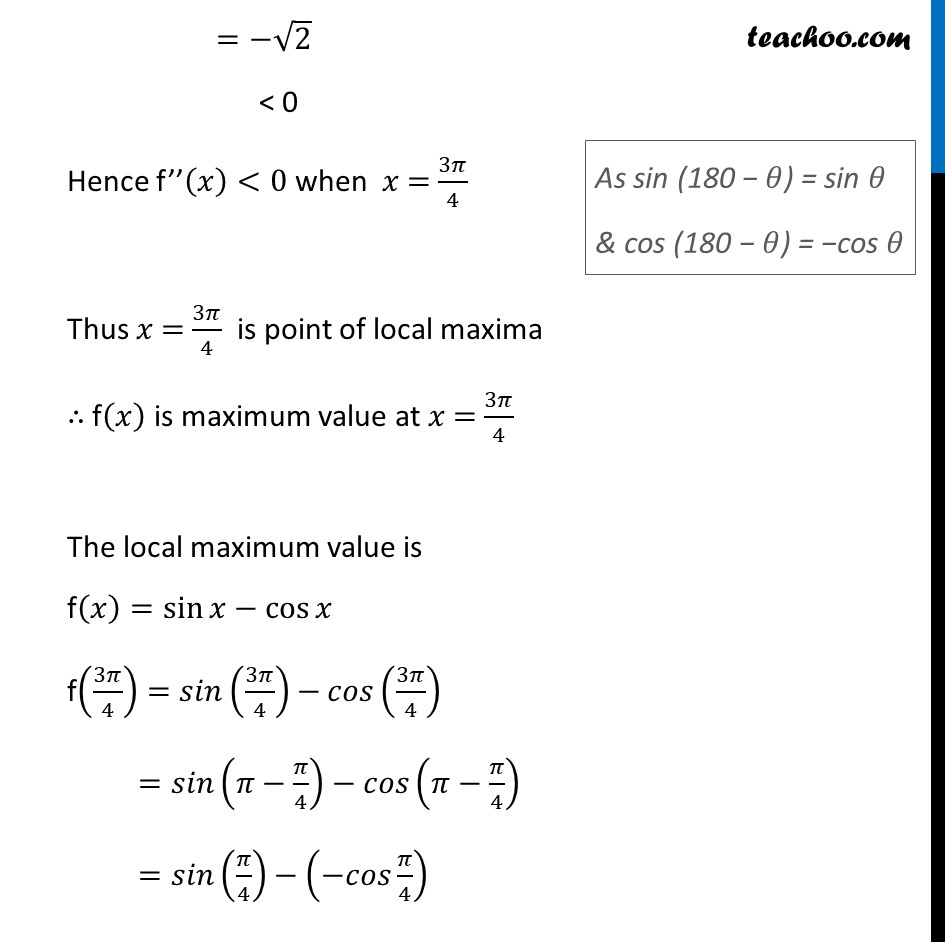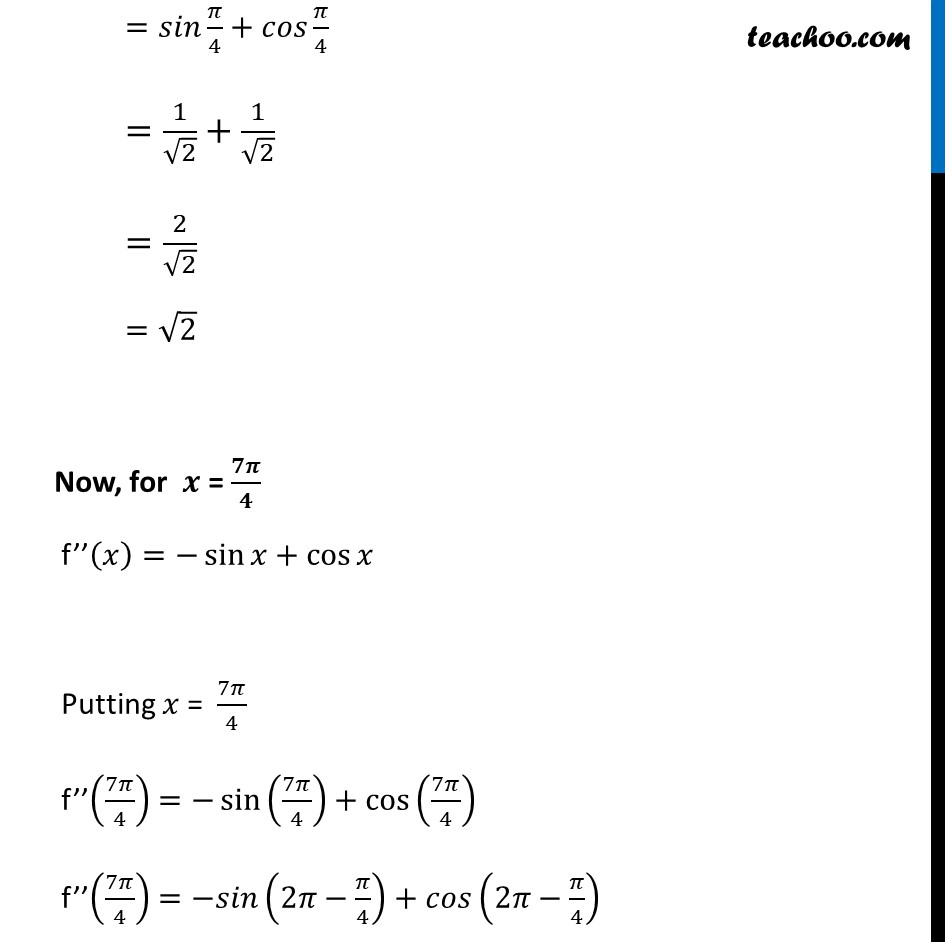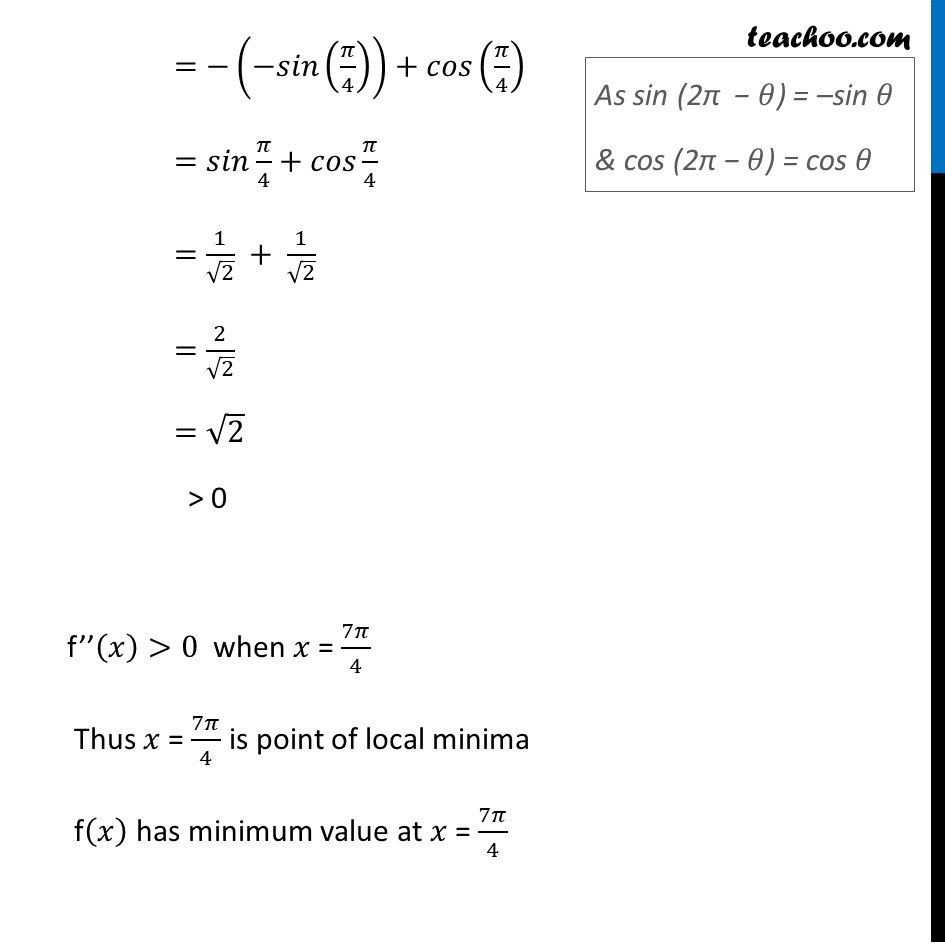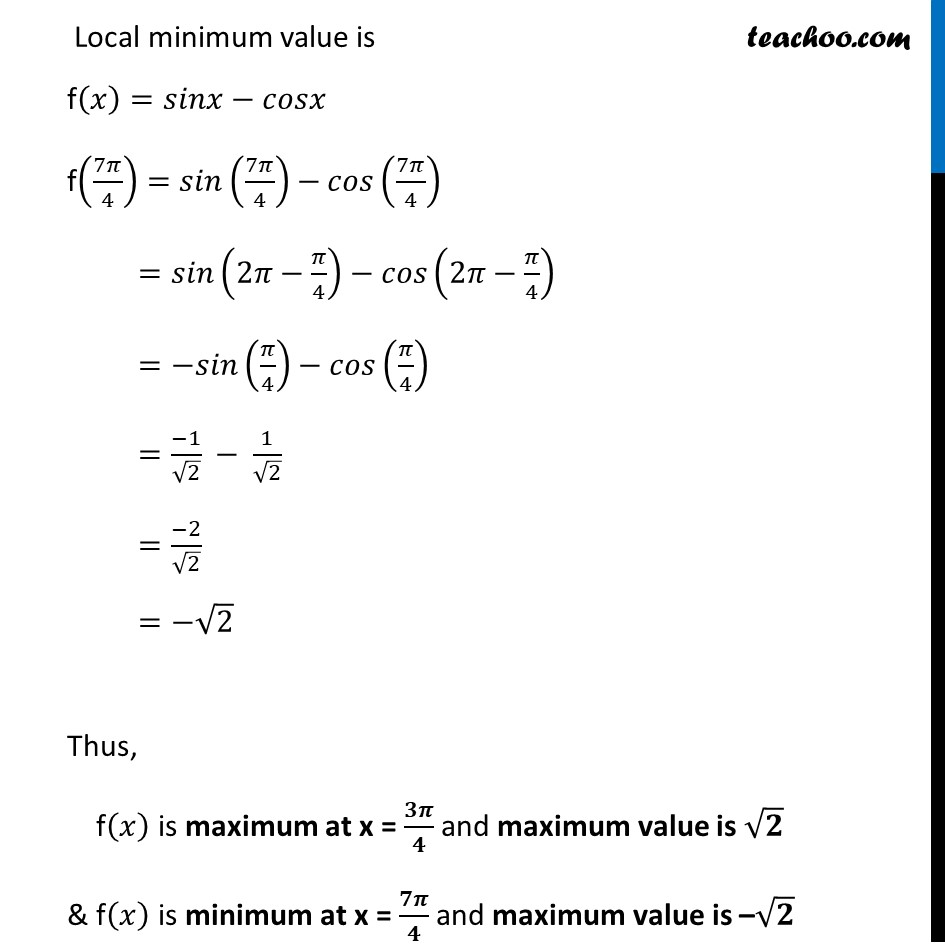Learn in your speed, with individual attention - Teachoo Maths 1-on-1 Class

### Transcript

Ex 6.3, 3 Find the local maxima and local minima, if any, of the following functions. Find also the local maximum and the local minimum values, as the case may be: (iv) f (𝑥)=sin⁡𝑥 –cos⁡𝑥, 0<𝑥<2 𝜋f (𝑥)=sin⁡𝑥 –cos⁡𝑥, 0<𝑥<2 𝜋 Finding f’(𝒙) f’(𝑥)=cos⁡𝑥−(−sin⁡𝑥 ) f’(𝑥)=cos⁡𝑥+sin⁡𝑥 Putting f’(𝒙)=𝟎 cos⁡𝑥+sin⁡𝑥 = 0 cos⁡𝑥=−sin⁡𝑥 1=(−sin⁡𝑥)/cos⁡𝑥 (−sin⁡𝑥)/cos⁡𝑥 =1 – tan 𝑥=1 tan 𝑥=−1 Since 0 < 𝑥 < 2π & tan 𝑥 is negative tan θ lies in either 2nd or 4th quadrant So, value of 𝑥 is 𝑥=3𝜋/4 𝑜𝑟 7𝜋/4 Now finding f’’(𝑥) f’’(𝑥)=𝑑(cos⁡𝑥 + sin⁡𝑥 )/𝑑𝑥 f’’(𝑥)=−sin⁡𝑥+cos⁡𝑥 Putting 𝒙 = 𝟑𝝅/𝟒 f’’(3𝜋/4)=−𝑠𝑖𝑛(3𝜋/4)+𝑐𝑜𝑠(3𝜋/4) =−𝑠𝑖𝑛(𝜋−𝜋/4)+𝑐𝑜𝑠(𝜋− 𝜋/4) =−𝑠𝑖𝑛(𝜋/4)+(−𝑐𝑜𝑠 𝜋/4) =(−1)/√2−1/√2 =(−2)/√2 =−√2 < 0 Hence f’’(𝑥)<0 when 𝑥 = 3𝜋/4 Thus 𝑥 = 3𝜋/4 is point of local maxima ∴ f(𝑥) is maximum value at 𝑥 = 3𝜋/4 The local maximum value is f(𝑥)=sin⁡𝑥−cos⁡𝑥 f(3𝜋/4)=𝑠𝑖𝑛(3𝜋/4)−𝑐𝑜𝑠(3𝜋/4) =𝑠𝑖𝑛(𝜋−𝜋/4)−𝑐𝑜𝑠(𝜋−𝜋/4) =𝑠𝑖𝑛(𝜋/4)−(−𝑐𝑜𝑠 𝜋/4) =𝑠𝑖𝑛 𝜋/4+𝑐𝑜𝑠 𝜋/4 =1/√2+1/√2 =2/√2 =√2 Now, for 𝒙 = 𝟕𝝅/𝟒 f’’(𝑥)=−sin⁡𝑥+cos⁡𝑥 Putting 𝑥 = 7𝜋/4 f’’(7𝜋/4)=−sin⁡(7𝜋/4)+cos⁡(7𝜋/4) f’’(7𝜋/4)=−𝑠𝑖𝑛(2𝜋−𝜋/4)+𝑐𝑜𝑠(2𝜋−𝜋/4) =−(−𝑠𝑖𝑛(𝜋/4))+𝑐𝑜𝑠(𝜋/4) =𝑠𝑖𝑛 𝜋/4+𝑐𝑜𝑠 𝜋/4 =1/√2 + 1/√2 =2/√2 =√2 > 0 f’’(𝑥)>0 when 𝑥 = 7𝜋/4 Thus 𝑥 = 7𝜋/4 is point of local minima f(𝑥) has minimum value at 𝑥 = 7𝜋/4 Local minimum value is f(𝑥)=𝑠𝑖𝑛𝑥−𝑐𝑜𝑠𝑥 f(7𝜋/4)=𝑠𝑖𝑛(7𝜋/4)−𝑐𝑜𝑠(7𝜋/4) =𝑠𝑖𝑛(2𝜋−𝜋/4)−𝑐𝑜𝑠(2𝜋−𝜋/4) =−𝑠𝑖𝑛(𝜋/4)−𝑐𝑜𝑠(𝜋/4) =(−1)/√2 − 1/√2 =(−2)/√2 =−√2 Thus, f(𝑥) is maximum at x = 𝟑𝝅/𝟒 and maximum value is √𝟐 & f(𝑥) is minimum at x = 𝟕𝝅/𝟒 and maximum value is –√𝟐